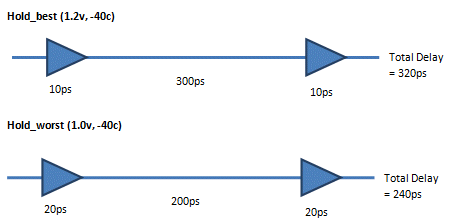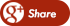﻿ Puzzle: Wire DelayBack

## Puzzle: Wire DelayTechnical Tidbit:

You have a long wire of let's say length L. And it has the net delay of 100ps. Now you split the wire into two equal halves of length 0.5L each and insert a buffer at the center of the wire. The delay of buffer is 25ps. What is the total delay of the system: (half wire+ buffer + half wire)?

Option a: 50+25+50= 125ps
Option b: 25+25+25=75ps
Option c: 25+25+50=100ps
Option d: 50+25+25=100ps

Choose the right option, and please give a short explanation.